# shortestpath

## 语法

``P = shortestpath(G,s,t)``
``P = shortestpath(G,s,t,'Method',algorithm)``
``[P,d] = shortestpath(___)``
``[P,d,edgepath] = shortestpath(___)``

## 说明

``P = shortestpath(G,s,t)` 计算从源节点 `s` 处开始到目标节点 `t` 处结束的最短路径。如果图进行了加权（即 `G.Edges` 包含变量 `Weight`），则这些权重用作沿图中各边的距离。否则，所有边距离都视为 `1`。`

``P = shortestpath(G,s,t,'Method',algorithm)` 可选择性地指定在计算最短路径时使用的算法。例如，如果 `G` 是加权图，则 `shortestpath(G,s,t,'Method','unweighted')` 将忽略 `G` 中的边权重，而将所有边权重视为 `1`。`

``[P,d] = shortestpath(___)` 支持上述语法中的任何输入参数，且可返回最短路径的长度 `d`。`

``[P,d,edgepath] = shortestpath(___)` 还返回从 `s` 到 `t` 的最短路径上所有边的边索引 `edgepath`。`

## 示例

```s = [1 1 2 3 3 4 4 6 6 7 8 7 5]; t = [2 3 4 4 5 5 6 1 8 1 3 2 8]; G = digraph(s,t); plot(G)```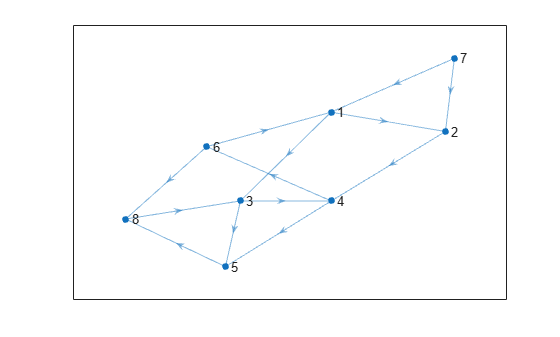`P = shortestpath(G,7,8)`
```P = 1×5 7 1 3 5 8 ```

```s = [1 1 1 2 2 6 6 7 7 3 3 9 9 4 4 11 11 8]; t = [2 3 4 5 6 7 8 5 8 9 10 5 10 11 12 10 12 12]; weights = [10 10 10 10 10 1 1 1 1 1 1 1 1 1 1 1 1 1]; G = graph(s,t,weights); plot(G,'EdgeLabel',G.Edges.Weight)```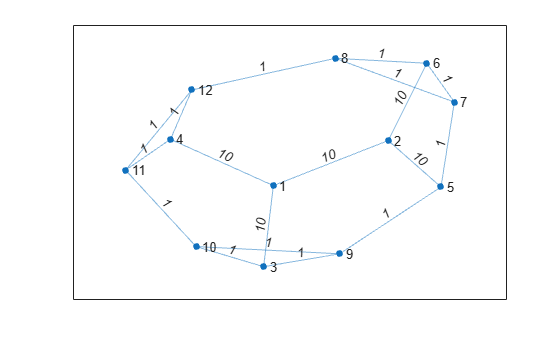`[P,d] = shortestpath(G,3,8)`
```P = 1×5 3 9 5 7 8 ```
```d = 4 ```

```s = [1 1 1 1 1 2 2 7 7 9 3 3 1 4 10 8 4 5 6 8]; t = [2 3 4 5 7 6 7 5 9 6 6 10 10 10 11 11 8 8 11 9]; weights = [1 1 1 1 3 3 2 4 1 6 2 8 8 9 3 2 10 12 15 16]; G = graph(s,t,weights); x = [0 0.5 -0.5 -0.5 0.5 0 1.5 0 2 -1.5 -2]; y = [0 0.5 0.5 -0.5 -0.5 2 0 -2 0 0 0]; p = plot(G,'XData',x,'YData',y,'EdgeLabel',G.Edges.Weight);```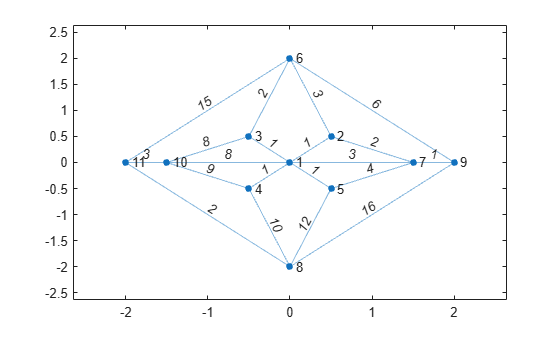`[path1,d] = shortestpath(G,6,8)`
```path1 = 1×5 6 3 1 4 8 ```
```d = 14 ```
`highlight(p,path1,'EdgeColor','g')`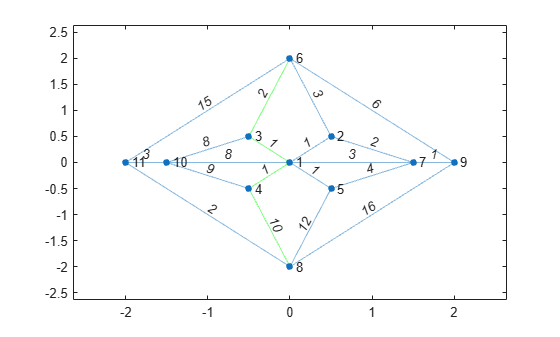`Method` 指定为 `unweighted` 以忽略边权重，转而将所有边的权重都视为 1。此方法会在节点之间生成一条不同路径，该路径以前因长度太大而不能成为最短路径。以红色突出显示此路径。

`[path2,d] = shortestpath(G,6,8,'Method','unweighted')`
```path2 = 1×3 6 9 8 ```
```d = 2 ```
`highlight(p,path2,'EdgeColor','r')`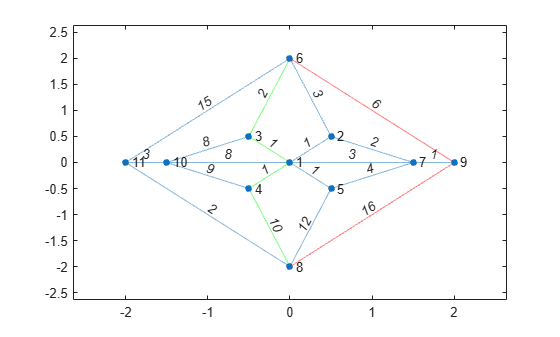```G = graph([1 1 1 1 1 2 2 3 3 3 4 4],[2 2 2 2 2 3 4 4 5 5 5 2],[2 4 6 8 10 5 3 1 5 6 8 9]); p = plot(G,'EdgeLabel',G.Edges.Weight);```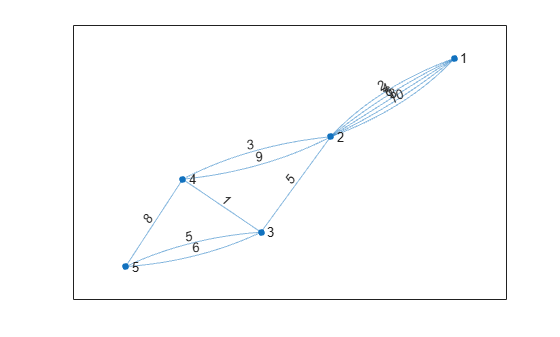`[P,d,edgepath] = shortestpath(G,1,5)`
```P = 1×5 1 2 4 3 5 ```
```d = 11 ```
```edgepath = 1×4 1 7 9 10 ```

`G.Edges(edgepath,:)`
```ans=4×2 table EndNodes Weight ________ ______ 1 2 2 2 4 3 3 4 1 3 5 5 ```

`highlight(p,'Edges',edgepath)`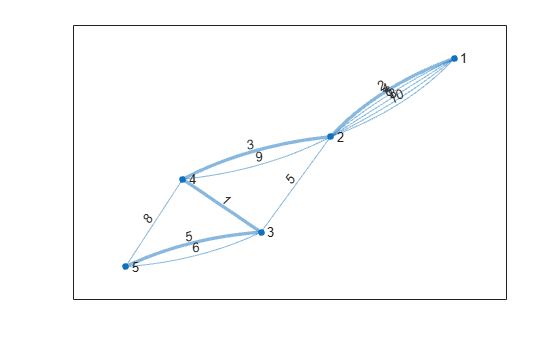```s = [1 1 2 2 3 4 4 4 5 5 6 6 7 8 9]; t = [2 4 3 5 6 5 7 9 6 7 7 8 9 10 10]; G = graph(s,t);```

```x = [1 2 3 2 2.5 4 3 5 3 5]; y = [1 3 4 -1 2 3.5 1 3 0 1.5]; plot(G,'XData',x,'YData',y)```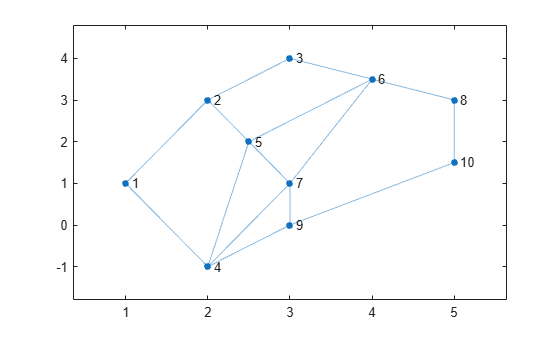`$\mathit{d}=\sqrt{{|\Delta \mathit{x}|}^{2}+{|\Delta \mathit{y}|}^{2}}=\sqrt{{|{\mathit{x}}_{\mathit{s}}-{\mathit{x}}_{\mathit{t}}|}^{2}+{|{\mathit{y}}_{\mathit{s}}-{\mathit{y}}_{\mathit{t}}|}^{2}}.$`

```[sn,tn] = findedge(G); dx = x(sn) - x(tn); dy = y(sn) - y(tn); D = hypot(dx,dy);```

```G.Edges.Weight = D'; p = plot(G,'XData',x,'YData',y,'EdgeLabel',G.Edges.Weight);```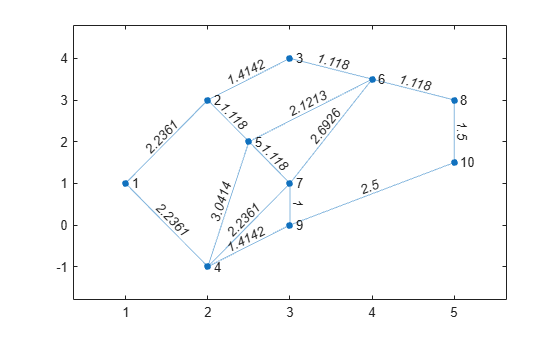`[path,len] = shortestpath(G,1,10)`
```path = 1×4 1 4 9 10 ```
```len = 6.1503 ```

`highlight(p,path,'EdgeColor','r','LineWidth',2)`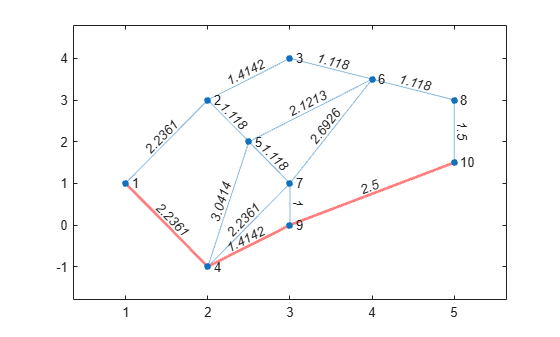## 输入参数

`'auto'`（默认值）

`'auto'` 选项会自动选择算法：

• `'unweighted'` 用于没有边权重的 `graph``digraph` 输入。

• `'positive'` 用于具有边权重的所有 `graph` 输入，并要求权重为非负数。此选项还用于具有非负边权重的 `digraph` 输入。

• `'mixed'` 用于其边权重包含某些负值的 `digraph` 输入。图不能包含负循环。

`'unweighted'`

`'positive'`

Dijkstra 算法，要求所有边权重均为非负数。

`'mixed'`（仅适用于 `digraph`

`'acyclic'`（仅适用于 `digraph`

## 输出参数

• 如果 `s``t` 包含数值节点索引，则 `P` 是节点索引的数值向量。

• 如果 `s``t` 包含节点名称，则 `P` 是包含节点名称的元胞数组或字符串数组。

## 提示

• `shortestpath``shortestpathtree``distances` 函数不支持具有负边权重的无向图，或更通俗地说，不支持包含负循环的任何图，原因如下：

• 负循环是从节点出发回到自身的路径，路径上的边权重之和为负值。如果两个节点之间的路径上具有负循环，则这两个节点之间不存在最短路径，因为始终可以通过遍历负循环找到更短路径。

• 无向图中的单个负边权重会创建一个负循环。